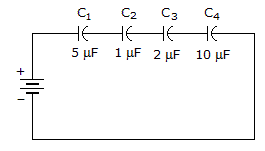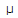# Electronics - Capacitors

### Exercise :: Capacitors - True or False

1.

Capacitive reactance is inversely proportional with frequency.

 A. True B. False

Explanation:

No answer description available for this question. Let us discuss.

2.

To find the total capacitance of capacitors in parallel, sum the values of the individual capacitors.

 A. True B. False

Explanation:

No answer description available for this question. Let us discuss.

3.The total capacitance in the given circuit is larger than the value of C4.

 A. True B. False

Explanation:

No answer description available for this question. Let us discuss.

4.

A capacitor is charged when one of its plates has more electrons than the other plate.

 A. True B. False

Explanation:

No answer description available for this question. Let us discuss.

5.

A 10F capacitor will have more capacitive reactance than a 20F capacitor in a 60 Hz circuit.

 A. True B. False